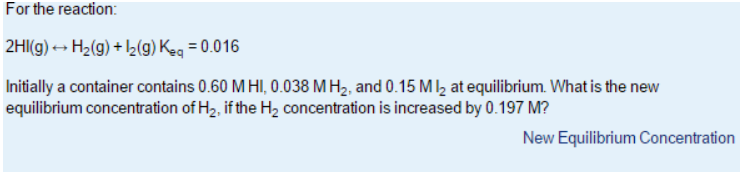# Problem: For the reaction 2HI (g) &lt;-&gt; H2 (g) + I2 (g)      Keq = 0.016 Initially a container contains 0.60 M HI, 0.038 M H 2, and 0.15 M I 2 at equilibrium. What is the new equilibrium concentration of H2, if the H2 concentration is increased by 0.197 M?

###### FREE Expert Solution
98% (315 ratings)###### Problem Details

For the reaction

2HI (g) <-> H2 (g) + I2 (g)      Keq = 0.016

Initially a container contains 0.60 M HI, 0.038 M H 2, and 0.15 M I 2 at equilibrium. What is the new equilibrium concentration of H2, if the H2 concentration is increased by 0.197 M?